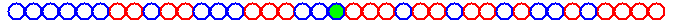My research is on the interface of probability theory and rigorous aspects of statistical mechanics.

I work on understanding the evolution of systems with complex interactions, such as particles moving in a disordered environment, cars navigating their way through traffic, the surface of a growing crystal, or the boundary of an infected tissue.

Complexity is captured by the randomness in the model, both in the environment in which the particles interact or the crystal grows, and in the interaction or growth process itself.

I develop the mathematical laws that govern such systems.

To have a very simple example in mind, think of how the fraction of Heads in a large number of tosses of a coin will converge to the probability of getting Heads in one toss. (The mathematical law behind this basic phenomenon is known as the Law of Large Numbers.)

Here are the slides from my Science Night Live public lecture explaining my research area.

One model I have studied is random walk in random environment.